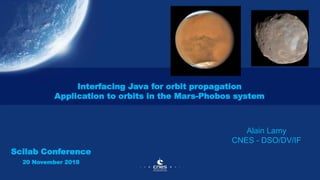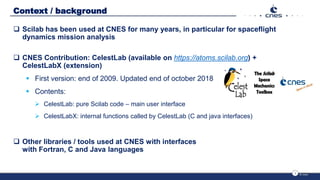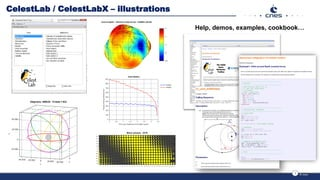Successfully reported this slideshow.

# CNES @ Scilab Conference 2018×

1 of 14
1 of 14

# CNES @ Scilab Conference 2018

Interfacing Java for orbit propagation
Application to orbits in the Mars-Phobos system

Interfacing Java for orbit propagation
Application to orbits in the Mars-Phobos system

### CNES @ Scilab Conference 2018

1. 1. Interfacing Java for orbit propagation Application to orbits in the Mars-Phobos system Alain Lamy CNES - DSO/DV/IF Scilab Conference 20 November 2018
2. 2. Context / background  Scilab has been used at CNES for many years, in particular for spaceflight dynamics mission analysis  CNES Contribution: CelestLab (available on https://atoms.scilab.org) + CelestLabX (extension)  First version: end of 2009. Updated end of october 2018  Contents:  CelestLab: pure Scilab code – main user interface  CelestLabX: internal functions called by CelestLab (C and java interfaces)  Other libraries / tools used at CNES with interfaces with Fortran, C and Java languages 2
3. 3. CelestLab / CelestLabX – illustrations 3 Help, demos, examples, cookbook…
4. 4. Why interfacing with other languages ?  For efficiency reasons  To make existing software available. Examples (CelestLabX):  STELA: long-term orbit propagation -> Java  TLEs: orbit data / model for propagation of various space objects -> C++  To be consistent with the original (same code)  Fewer bugs. Less testing effort (interfaced code may be complex)  But additional effort necessary… has to be worth it  To attract new users interested in the additional features. 4
5. 5. Example of use of Java interface (STELA)  Example from Celestlab // Initial date (time scale: TREF) cjd0 = CL_dat_cal2jd(2018, 11, 16); // Keplerian mean orbital elements (frame: ECI) mean_kep0 = [7.e6; 1.e-3; 98*(%pi/180); %pi/2; 0; 0]; // Final dates cjd = cjd0 + (0:60); // STELA model parameters (default values) params = CL_stela_params(); // Propagation mean_kep = CL_stela_extrap("kep", cjd0, mean_kep0, cjd, params, "m"); // Plot inclination (deg) scf(); plot(cjd – cjd0, mean_kep(3,:) * (180/%pi)); CL_g_stdaxes(); Structure of model parameters (forces…) Initial state Extrapolation dates Easy to use Looks like pure Scilab code 5
6. 6. Numerical integration of orbital motion  Position = ‫׭‬ σ 𝒂𝒄𝒄𝒆𝒍𝒆𝒓𝒂𝒕𝒊𝒐𝒏  Several examples in CelestLab using ode  Rather straightforward, but the computation of some forces can be very time consuming => slow execution  One possibility: interface functions written in compiled languages => would imply lots of rewriting. 6 // Dynamic model function [ydot]=fct(t, y) pos = y(1:3,:); vel = y(4:6,:); ydot = zeros(y); ydot(1:3,:) = vel; ydot(4:6,:) = CL_fo_centralAcc(pos); endfunction // Initial state t0 = 0; y0 = [7.e6; 0; 0; 0; 7.e3; 0]; t = (0:180:10000); // time instants (seconds) // Integration rtol = 1.e-12 * [1;1;1;1;1;1]; atol = 1.e-6 * [1;1;1;1.e-3;1.e-3;1.e-3]; y = ode(y0, t0, t, rtol, atol, fct);
7. 7. Other solution: interface Java  Why Java ?  Library for flight dynamics software exists (used in future control centers)  Called Patrius (in addition: freeware: see https://logiciels.cnes.fr/en)  Contains lots of features that enable the computation of trajectories => Idea: Design an interface for numerical propagation similar to what already exists for STELA 7
8. 8. Basic example  Typical example (from function help) 8 // Initial orbit state (position/velocity, frame: CIRS, time scale: TREF) cjd0 = CL_dat_cal2cjd(2004, 10, 4); kep0 = [7.e6; 1.e-3; 98 * (%pi/180); 0; 0; 0]; pv0 = CL_oe_convert("kep", "pv", kep0); // Epoch of final orbit states cjd = cjd0 + (0 : 60 : 86400) / 86400; // Model parameters (default values) params = ms_orbp_params(); // Propagation pv = ms_orbp_extrap(cjd0, pv0, cjd, params, "pv"); // Plot osculating semi-major axis and inclination kep = CL_oe_convert("pv", "kep", pv); scf(); plot(cjd, kep(1,:) / 1000); xtitle("Semi major axis (km)"); Similar to STELA example Looks like pure Scilab code Easy to use
9. 9. Features  Simple interfaces  Computation of position / velocity / acceleration at selected dates, and also of:  Jacobian matrix at each date (hypermatrix 6x6xN) : 𝜕𝑝𝑣 𝜕𝑝𝑣0  Derivative at each date wrt selected parameters (matrix 6xN) : 𝜕𝑝𝑣 𝜕𝑝 => can be used in more complex applications: orbit adjustment… Design aspect: Scilab code for the interface: as simple as possible. As much as possible is done in specific java interface code. 9
10. 10. Application: Mars – Phobos system  Mars  Average distance from the Sun: 1.5 AU  Radius: about 3400 km  Phobos :  One of the 2 satellites of Mars  Size: 26x22x18 km  Distance from Mars center: < 3 Mars radii  Orbits: QSO (quasi satellite orbits) around Mars / close to Phobos 10
11. 11. Typical studies that have been conducted  Theoretical study of QSOs with simplified model (=> particular properties)  Comparison with more accurate models  Adjustment of initial state (least squares)  Multiple shooting (multiple adjustments on consecutive arcs)  etc… 11
12. 12. Illustrations (results)  Propagation from almost arbitrary initial conditions 12 Inertial frame (km) Rotating frame (km) Trajectory very sensitive to initial conditions (yellow dots: collision with Phobos)
13. 13. Conclusion  Main points  Interfacing Java and Scilab is rather easy  No compiled code => no additional tools needed (compilers…)  Possible limitation: incompatible Java libraries in javaclasspath  CNES applications : STELA, numerical extrapolation as well as other simpler functions :  Very satisfying results  Tools have been used in projects under study (Mars – Phobos system, among others)  We’ll continue in this direction ! 13
14. 14. Annex  Scilab-Java interface: one remark  Example : 14 function F() jimport fr.cnes.xxx.Extrapolation; jextrapolation = jnewInstance(Extrapolation); … jremove(Extrapolation); jremove(jextrapolation); endfunction jremove necessary otherwise: memory leaks… Very tedious and not safe in case of errors – similar to freeing memory in C => Improvements would be worthwhile